SNVS663H June   2010  – August 2015

PRODUCTION DATA.

1. Features
2. Applications
3. Description
4. Revision History
5. Pin Configuration and Functions
6. Specifications
7. Detailed Description
1. 7.1 Overview
2. 7.2 Functional Block Diagram
3. 7.3 Feature Description
4. 7.4 Device Functional Modes
8. Application and Implementation
1. 8.1 Application Information
2. 8.2 Typical Application
1. 8.2.1 Design Requirements
2. 8.2.2 Detailed Design Procedure
3. 8.2.3 Application Curves
9. Power Supply Recommendations
10. 10Layout
11. 11Device and Documentation Support
1. 11.1 Device Support
2. 11.2 Documentation Support
3. 11.3 Community Resources
5. 11.5 Electrostatic Discharge Caution
6. 11.6 Glossary
12. 12Mechanical, Packaging, and Orderable Information

• NDW|7

## 8 Application and Implementation

NOTE

Information in the following applications sections is not part of the TI component specification, and TI does not warrant its accuracy or completeness. TI’s customers are responsible for determining suitability of components for their purposes. Customers should validate and test their design implementation to confirm system functionality.

### 8.1 Application Information

The LMZ12003EXT is a step-down DC-to-DC power module. It is typically used to convert a higher DC voltage to a lower DC voltage with a maximum output current of 3 A. The following design procedure can be used to select components for the LMZ12003EXT. Alternately, the WEBENCH software may be used to generate complete designs.

When generating a design, the WEBENCH software utilizes iterative design procedure and accesses comprehensive databases of components. Please go to www.ti.com for more details

### 8.2 Typical Application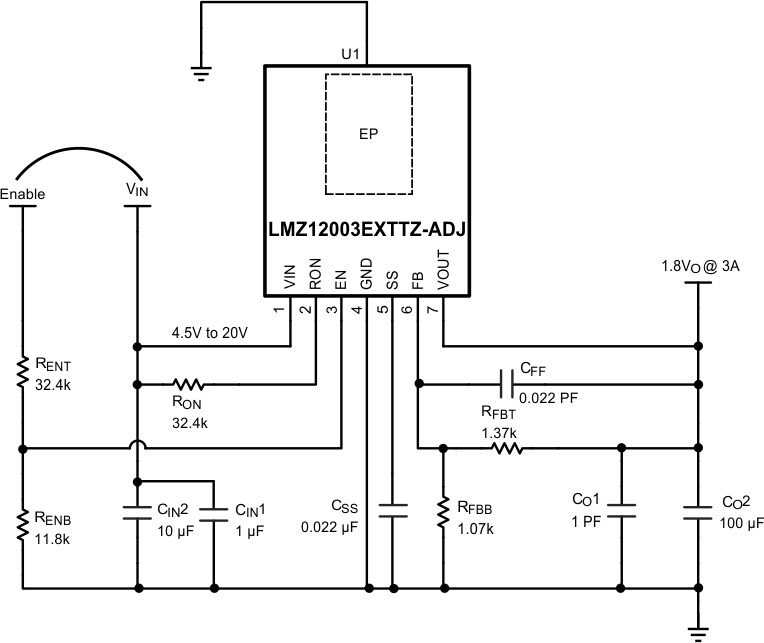Figure 21. Evaluation Board Schematic Diagram

#### 8.2.1 Design Requirements

For this example the following application parameters exist.

• VIN Range = Up to 20 V
• VOUT = 0.8 V to 6 V
• IOUT = 3 A

#### 8.2.2 Detailed Design Procedure

The LMZ12003EXT is fully supported by WEBENCH and offers the following: Component selection, electrical and thermal simulations as well as the build-it board for a reduction in design time. The following list of steps can be used to manually design the LMZ12003EXT application.

1. Select minimum operating VIN with enable divider resistors
2. Program VO with divider resistor selection
3. Program turnon time with soft-start capacitor selection
4. Select CO
5. Select CIN
6. Set operating frequency with RON
7. Determine module dissipation
8. Layout PCB for required thermal performance

#### 8.2.2.1 Enable Divider, RENT and RENB Selection

The enable input provides a precise 1.18-V band-gap rising threshold to allow direct logic drive or connection to a voltage divider from a higher enable voltage such as Vin. The enable input also incorporates 90 mV (typical) of hysteresis resulting in a falling threshold of 1.09 V. The maximum recommended voltage into the EN pin is 6.5 V. For applications where the midpoint of the enable divider exceeds 6.5 V, a small Zener can be added to limit this voltage.

The function of this resistive divider is to allow the designer to choose an input voltage below which the circuit will be disabled. This implements the feature of programmable undervoltage lockout. This is often used in battery powered systems to prevent deep discharge of the system battery. It is also useful in system designs for sequencing of output rails or to prevent early turnon of the supply as the main input voltage rail rises at power-up. Applying the enable divider to the main input rail is often done in the case of higher input voltage systems where a lower boundary of operation must be established. In the case of sequencing supplies, the divider is connected to a rail that becomes active earlier in the power-up cycle than the LMZ12003EXT output rail. The two resistors must be chosen based on the following ratio:

Equation 1. RENT / RENB = (VIN UVLO / 1.18 V) – 1

The LMZ12003EXT demonstration and evaluation boards use 11.8 kΩ for RENB and 32.4 kΩ for RENT resulting in a rising UVLO of 4.5 V. This divider presents 5.34 V to the EN input when the divider input is raised to 20 V.

#### 8.2.2.2 Output Voltage Selection

Output voltage is determined by a divider of two resistors connected between VO and ground. The midpoint of the divider is connected to the FB input. The voltage at FB is compared to a 0.8-V internal reference. In normal operation an ON-time cycle is initiated when the voltage on the FB pin falls below 0.8 V. The main MOSFET ON-time cycle causes the output voltage to rise and the voltage at the FB to exceed 0.8 V. As long as the voltage at FB is above 0.8 V, ON-time cycles will not occur.

The regulated output voltage determined by the external divider resistors RFBT and RFBB is:

Equation 2. VO = 0.8 V × (1 + RFBT / RFBB)

Rearranging terms; the ratio of the feedback resistors for a desired output voltage is:

RFBT / RFBB = (VO / 0.8 V) – 1

These resistors must be chosen from values in the range of 1.0 kΩ to 10.0 kΩ.

For VO = 0.8 V the FB pin can be connected to the output directly so long as an output preload resistor remains that draws more than 20 µA. Converter operation requires this minimum load to create a small inductor ripple current and maintain proper regulation when no load is present.

A feed-forward capacitor is placed in parallel with RFBT to improve load step transient response. Its value is usually determined experimentally by load stepping between DCM and CCM conduction modes and adjusting for best transient response and minimum output ripple.

Table 1 lists the values for RFBT , RFBB , CFF and RON.

### Table 1. Bill of Materials

REF DES DESCRIPTION CASE SIZE MANUFACTURER MANUFACTURER P/N
U1 SIMPLE SWITCHER PFM-7 Texas Instruments LMZ12003EXTTZ-ADJ
Cin1 1 µF, 50 V, X7R 1206 Taiyo Yuden UMK316B7105KL-T
Cin2 10 µF, 50 V, X7R 1210 Taiyo Yuden UMK325BJ106MM-T
CO1 1 µF, 50 V, X7R 1206 Taiyo Yuden UMK316B7105KL-T
CO2 100 µF, 6.3 V, X7R 1210 Taiyo Yuden JMK325BJ10CR7MM-T
RFBT 1.37 kΩ 0603 Vishay Dale CRCW06031K37FKEA
RFBB 1.07 kΩ 0603 Vishay Dale CRCW06031K07FKEA
RON 32.4 kΩ 0603 Vishay Dale CRCW060332K4FKEA
RENT 32.4 kΩ 0603 Vishay Dale CRCW060332K4FKEA
RENB 11.8 kΩ 0603 Vishay Dale CRCW060311k8FKEA
CFF 22 nF, ±10%, X7R, 16 V 0603 TDK C1608X7R1H223K
CSS 22 nF, ±10%, X7R, 16 V 0603 TDK C1608X7R1H223K

#### 8.2.2.3 Soft-Start Capacitor Selection

Programmable soft-start permits the regulator to slowly ramp to its steady-state operating point after being enabled, thereby reducing current inrush from the input supply and slowing the output voltage rise-time to prevent overshoot.

Upon turnon, after all UVLO conditions have been passed, an internal 8-µA current source begins charging the external soft-start capacitor. The soft-start time duration to reach steady-state operation is given by the formula:

Equation 3. tSS = VREF × CSS / Iss = 0.8 V × CSS / 8 µA

This equation can be rearranged as follows:

CSS = tSS × 8 μA / 0.8 V

Use of a 0.022-μF capacitor results in 2.2-ms soft-start duration. This is recommended as a minimum value.

As the soft-start input exceeds 0.8 V the output of the power stage will be in regulation. The soft-start capacitor continues charging until it reaches approximately 3.8 V on the SS pin. Voltage levels between 0.8 V and 3.8 V have no effect on other circuit operation. The following conditions will reset the soft-start capacitor by discharging the SS input to ground with an internal 200-μA current sink.

• The enable input being pulled low

• Thermal shutdown condition

• Overcurrent fault

• Internal VCC UVLO (Approx 4-V input to VIN)

#### 8.2.2.4 CO Selection

None of the required CO output capacitance is contained within the module. At a minimum, the output capacitor must meet the worst case minimum ripple current rating of 0.5 × ILR P-P, as calculated in Equation 14. Beyond that, additional capacitance will reduce output ripple so long as the ESR is low enough to permit it. A minimum value of 10 μF is generally required. Experimentation will be required if attempting to operate with a minimum value. Ceramic capacitors or other low ESR types are recommended. See AN-2024 SNVA422 for more detail.

The following equation provides a good first pass approximation of CO for load transient requirements:

Equation 4. CO ≥ ISTEP × VFB × L × VIN / (4 × VO × (VIN – VO) × VOUT-TRAN)

Solving:

CO ≥ 3 A × 0.8 V × 6.8 μH × 12 V / (4 × 3.3 V × (12 V – 3.3 V) × 33 mV)
≥ 52μF

The LMZ12003EXT demonstration and evaluation boards are populated with a 100-µF 6.3-V X5R output capacitor. Locations for extra output capacitors are provided.

#### 8.2.2.5 CIN Selection

The LMZ12003EXT module contains an internal 0.47-µF input ceramic capacitor. Additional input capacitance is required external to the module to handle the input ripple current of the application. This input capacitance must be located in very close proximity to the module. Input capacitor selection is generally directed to satisfy the input ripple current requirements rather than by capacitance value. Worst case input ripple current rating is dictated by the equation:

Equation 5. I(CIN(RMS)) ≊ 1 /2 × IO * × √ (D / 1-D)

where

• D ≊ VO / VIN

(As a point of reference, the worst case ripple current will occur when the module is presented with full load current and when VIN = 2 × VO).

Recommended minimum input capacitance is 10-µF X7R ceramic with a voltage rating at least 25% higher than the maximum applied input voltage for the application. It is also recommended that attention be paid to the voltage and temperature deratings of the capacitor selected.

NOTE

Ripple current rating of ceramic capacitors may be missing from the capacitor data sheet and you may have to contact the capacitor manufacturer for this rating.

If the system design requires a certain minimum value of input ripple voltage ΔVIN be maintained then the following equation may be used.

Equation 6. CIN ≥ IO × D × (1 – D) / fSW-CCM × ΔVIN

If ΔVIN is 1% of VIN for a 20V input to 3.3-V output application this equals 200 mV and fSW = 400 kHz.

CIN ≥ 3 A × 3.3 V / 20 V × (1 – 3.3 V / 20 V) / (400000 × 0.200 V)
≥ 5.2 μF

Additional bulk capacitance with higher ESR may be required to damp any resonant effects of the input capacitance and parasitic inductance of the incoming supply lines.

#### 8.2.2.6 RON Resistor selection

Many designs will begin with a desired switching frequency in mind. For that purpose the following equation can be used.

Equation 7. fSW(CCM) ≊ VO / (1.3 × 10-10 × RON)

This can be rearranged as

Equation 8. RON ≊ VO / (1.3 × 10-10 × fSW(CCM))

The selection of RON and fSW(CCM) must be confined by limitations in the ON-time and OFF-time for the COT control section.

The ON-time of the LMZ12003EXT timer is determined by the resistor RON and the input voltage VIN. It is calculated as follows:

Equation 9. tON = (1.3 × 10-10 × RON) / VIN

The inverse relationship of tON and VIN gives a nearly constant switching frequency as VIN is varied. RON must be selected such that the ON-time at maximum VIN is greater than 150 ns. The ON-timer has a limiter to ensure a minimum of 150 ns for tON. This limits the maximum operating frequency, which is governed by the following equation:

Equation 10. fSW(MAX) = VO / (VIN(MAX) × 150 ns)

This equation can be used to select RON if a certain operating frequency is desired so long as the minimum ON-time of 150 ns is observed. The limit for RON can be calculated as follows:

Equation 11. RON ≥ VIN(MAX) × 150 ns / (1.3 × 10 -10)

If RON calculated in Equation 8 is less than the minimum value determined in Equation 11 a lower frequency must be selected. Alternatively, VIN(MAX) can also be limited in order to keep the frequency unchanged.

Additionally, consider the minimum OFF-time of 260 ns limits the maximum duty ratio. Larger RON (lower FSW) must be selected in any application requiring large duty ratio.

#### 8.2.2.6.1 Discontinuous Conduction and Continuous Conduction Modes Selection

Operating frequency in DCM can be calculated as follows:

Equation 12. fSW(DCM) ≊ VO × (VIN – 1) × 6.8 μH × 1.18 × 1020 × IO / (VIN – VO) × RON2

In CCM, current flows through the inductor through the entire switching cycle and never falls to zero during the OFF-time. The switching frequency remains relatively constant with load current and line voltage variations. The CCM operating frequency can be calculated using Equation 7 above.

Figure 22 is a comparison pair of waveforms of the showing both CCM (upper) and DCM operating modes.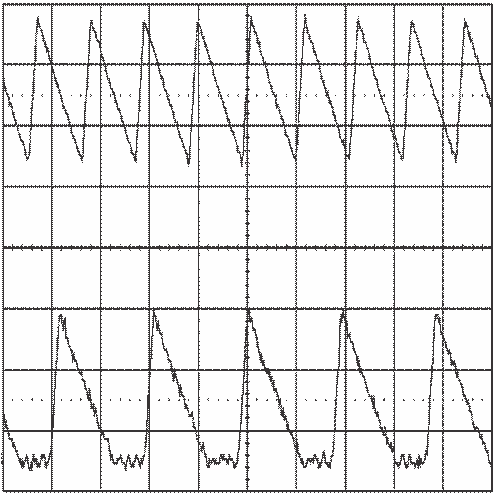VIN = 12 V, VO = 3.3 V, IO = 3 A /0.4 A 2 μs/div
Figure 22. CCM and DCM Operating Modes

The approximate formula for determining the DCM/CCM boundary is as follows:

Equation 13. IDCB ≊ VO × (VIN – VO) / (2 × 6.8 μH × fSW(CCM) × VIN)

Figure 23 is a typical waveform showing the boundary condition.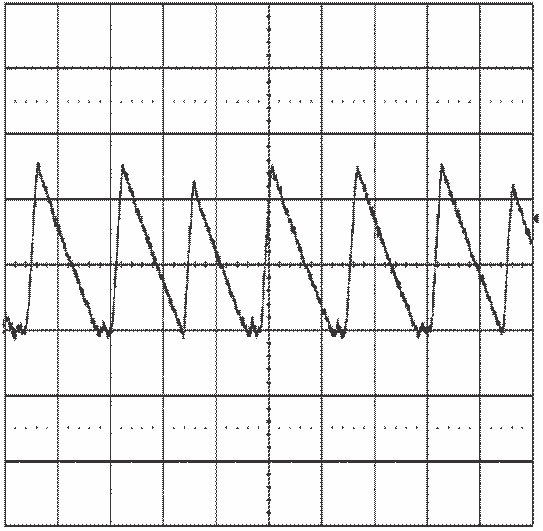VIN = 12 V, VO = 3.3 V, IO = 0.5 A 2 μs/div
Figure 23. Transition Mode Operation

The inductor internal to the module is 6.8 μH. This value was chosen as a good balance between low and high input voltage applications. The main parameter affected by the inductor is the amplitude of the inductor ripple current (ILR). ILR can be calculated with:

Equation 14. ILR P-P = VO × (VIN – VO) / (6.8 µH × fSW × VIN)

where

• VIN is the maximum input voltage
• and fSW is determined from Equation 7.

If the output current IO is determined by assuming that IO = IL, the higher and lower peak of ILR can be determined. Be aware that the lower peak of ILR must be positive if CCM operation is required.

#### 8.2.3 Application Curves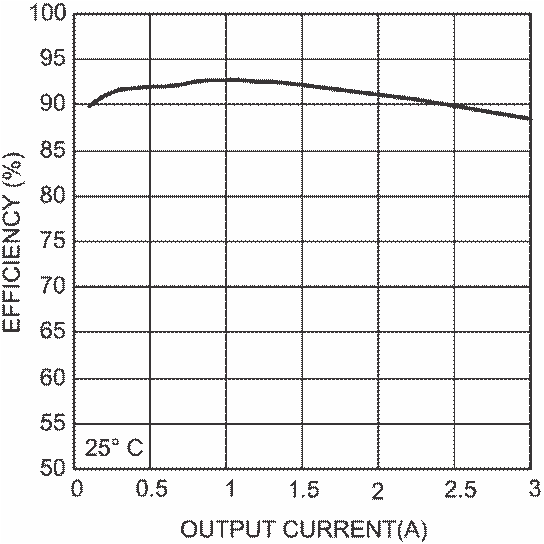VIN = 12 V, VOUT = 5 V
Figure 24. Efficiency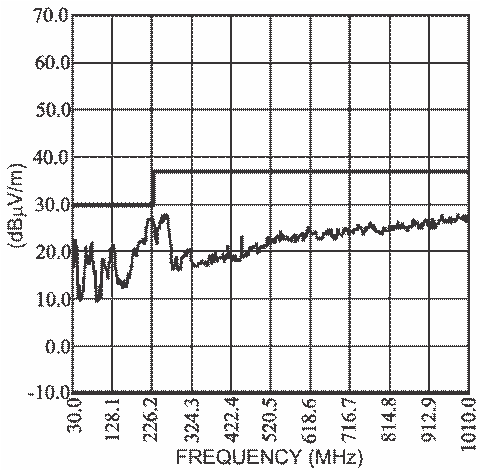Figure 26. Radiated Emissions (EN 55022 Class B)
from Evaluation Board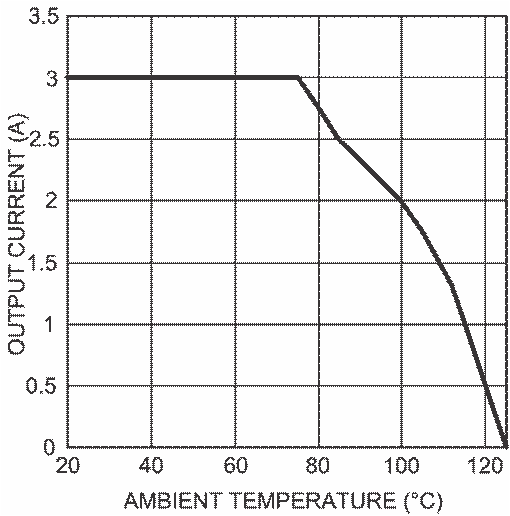VIN = 12 V, VOUT = 5 V
Figure 25. Thermal Derating Curve